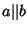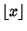## Concatenation

The concatenation of two stringsandis the stringformed by joiningand. Thus the concatenation of the strings book'' and case'' is the string bookcase''. The concatenation of two stringsandis often denoted,, or, in Mathematica(Wolfram Research, Champaign, IL),. Concatenation is an associative operation, so that the concatenation of three or more strings, for example,, etc., is well-defined.

The concatenation of two or more numbers is the number formed by concatenating their numerals. For example, the concatenation of 1, 234, and 5678 is 12345678. The value of the result depends on the numeric base, which is typically understood from context.

The formula for the concatenation of numbersandin baseiswhereis the Length ofin baseandis the Floor Function.